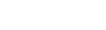﻿ Modifying DataMyDAC

# Modifying DataThis tutorial describes how to modify data in tables using the TMyQuery and TMyTable components.

## Requirements

This walkthrough supposes that you know how to connect to server (tutorials "Connecting To MySQL" and "Connecting To MySQL Embedded"), how to create necessary objects on the server (tutorial "Creating Database Objects"), and how to insert data to created tables (tutorial "Inserting Data Into Tables").

## General information

Data on server can be modified using Data Manipulation Language (DML), which is a part of SQL. DML statements can be executed on server by an account that has necessary privileges. There are two ways to manipulate a database. You can build DML statements manually and run them within some component like TMyQuery. Another way is to use the dataset functionality (the Edit and Post methods) of the TMyQuery and TMyTable components. We will discuss both ways. The goal of this tutorial is to modify the following record of the table dept:

 10 ACCOUNTING NEW YORK

to make it look as follows:

 10 RESEARCH LOS ANGELES

## Using DataSet Functionality

The Edit and Post methods of the TMyQuery and TMyTable components allow deleting data without using DML statements. DML statements are generated by MyDAC components internally. The code below demonstrates using these methods:

[Delphi]

``````var
q: TMyQuery;
begin
q := TMyQuery.Create(nil);
try
// con is either TMyConnection or TMyEmbConnection already set up
q.Connection := con;

// retrieve data
q.SQL.Text := 'SELECT * FROM dept';
q.Open;

// to make the record with deptno=10 the current record
q.FindKey();

// modify record
q.Edit;
q.FieldByName('dname').AsString := 'RESEARCH';
q.FieldByName('loc').AsString := 'LOS ANGELES';
q.Post;
finally
q.Free;
end;
end;``````

[C++Builder]

``````{
TMyQuery* q = new TMyQuery(NULL);
try
{
// con is either TMyConnection or TMyEmbConnection already set up
q->Connection = con;

// retrieve data
q->SQL->Text = "SELECT * FROM dept";
q->Open();

// to make the record with deptno=10 the current record
q->FindKey(ARRAYOFCONST((10)));

// modify record
q->Edit();
q->FieldByName("dname")->AsString = "RESEARCH";
q->FieldByName("loc")->AsString = "LOS ANGELES";
q->Post();
}
__finally
{
q->Free();
}
}``````

### Building DML Statements Manually

DML Statements can contain plain text and text with parameters. This section describes both ways.

### DML Statements With Parameters

[Delphi]

``````var
q: TMyQuery;
begin
q := TMyQuery.Create(nil);
try
// con is either TMyConnection or TMyEmbConnection already set up
q.Connection := con;

// set SQL query for update record
q.SQL.Clear;
q.SQL.Add('UPDATE dept SET dname = :dname, loc = :loc WHERE deptno = :deptno;');

// set parameters
q.ParamByName('deptno').AsInteger := 10;
q.ParamByName('dname').AsString := 'RESEARCH';
q.ParamByName('loc').AsString := 'LOS ANGELES';

// execute query
q.Execute;
finally
q.Free;
end;
end;``````

[C++Builder]

``````{
TMyQuery* q = new TMyQuery(NULL);
try
{
// con is either TMyConnection or TMyEmbConnection already set up
q->Connection = con;

// set SQL query for update record
q->SQL->Clear();
q->SQL->Add("UPDATE dept SET dname = :dname, loc = :loc WHERE deptno = :deptno;");

// set parameters
q->ParamByName("deptno")->AsInteger = 10;
q->ParamByName("dname")->AsString = "RESEARCH";
q->ParamByName("loc")->AsString = "LOS ANGELES";

// execute query
q->Execute();
}
__finally
{
q->Free();
}
}``````

### DML Statements As Plain Text

[Delphi]

``````var
q: TMyQuery;
begin
q := TMyQuery.Create(nil);
try
// con is either TMyConnection or TMyEmbConnection already set up
q.Connection := con;

// set SQL query for update record
q.SQL.Clear;
q.SQL.Add('UPDATE dept SET dname = ''RESEARCH'', loc = ''LOS ANGELES'' WHERE deptno = 10;');

// execute query
q.Execute;
finally
q.Free;
end;
end;``````

[C++Builder]

``````{
TMyQuery* q = new TMyQuery(NULL);
try
{
// con is either TMyConnection or TMyEmbConnection already set up
q->Connection = con;

// set SQL query for update record
q->SQL->Clear();
q->SQL->Add("UPDATE dept SET dname = 'RESEARCH', loc = 'LOS ANGELES' WHERE deptno = 10;");

// execute query
q->Execute();
}
__finally
{
q->Free();
}
}``````